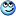## Main Question or Discussion Point

why $$1^\infty$$ is indeterminate form?

Mark44
Mentor
why $$1^\infty$$ is indeterminate form?
1 raised to any finite power is 1, of course, but some limits are of this indeterminate form, and have a limit that isn't equal to 1.

The most famous example is this limit:
$$\lim_{x \to \infty}(1 + \frac{1}{x})^x$$

The base is approaching 1 and the exponent is "approaching" infinity. It can be shown that the value of this limit expression is the number e.

Thanks ,, I got it now
(:

PeroK
Homework Helper
Gold Member
why $$1^\infty$$ is indeterminate form?
Anything with $\infty$ is an indeterminate form, because $\infty$ is not a number.

Mentallic
Homework Helper
Anything with $\infty$ is an indeterminate form, because $\infty$ is not a number.
I don't believe that $1/\infty$ is an indeterminate form because its value cannot be anything other than 0.

Mark44
Mentor
I don't believe that $1/\infty$ is an indeterminate form because its value cannot be anything other than 0.
I agree. It's not indeterminate because you can determine what the limit will be.

Mark44
Mentor
Anything with $\infty$ is an indeterminate form, because $\infty$ is not a number.
That's not true. While $[\infty - \infty]$ and $[\frac{\infty}{\infty}]$ are indeterminate forms, $[\infty + \infty]$ and $[\infty * \infty]$ are not considered indeterminate.

•Maged Saeed
That's not true. While $[\infty - \infty]$ and $[\frac{\infty}{\infty}]$ are indeterminate forms, $[\infty + \infty]$ and $[\infty * \infty]$ are not considered indeterminate.
I agree. It's not indeterminate because you can determine what the limit will be.
Me too .

$$\frac{0}{\infty}$$

Is it indeterminate too?

I think soMark44
Mentor
Me too .

$$\frac{0}{\infty}$$

Is it indeterminate too?

I think soNo, it is not indeterminate. If the numerator approaches 0 and the denominator becomes unbounded, the limit is 0.

•Maged Saeed
Oh, I see,,
Thanks